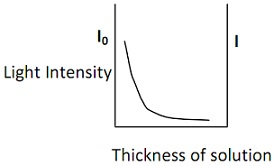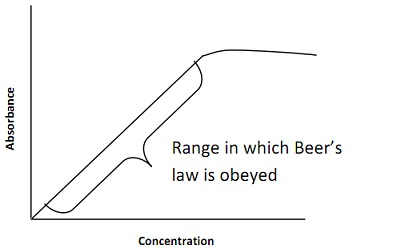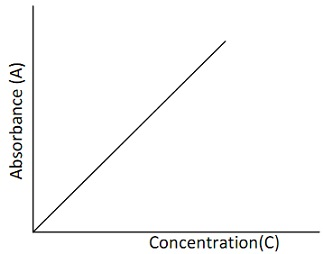#### Spectrochemical optical methods, Chemistry tutorial

Introduction

Optical process of analysis engages the measurement of light intensity. To relate optical measurement to the amount of a particular material in an example, it is needed to exploit the ability of atoms or molecules to absorb or emit light radiation whenever exposed to a particular set of conditions. Therefore this technique of analysis involves utilize of instrument through fluctuating degrees of sophistication.

Definition

Optical processes are spectroscopic techniques that are depends on the absorption or emission of ultraviolet, visible and infrared radiation.

Types of optical methods of analysis

• Colorimetry that involves the measurement of the absorption of light radiation through a coloured solution.
• Spectrophotometry which measures the absorption of light of a narrow wavelength band via molecules in solution.
• Atomic absorption analysis that calculates the absorption light radiation via free atomic specie.
• Flourimetry which engages the estimation of the amount of fluorescent substance in a following example.
• Atomic emission analysis that depend on the emission light atoms in exited electronic state.

Molecular absorption analysis

The absorption of light via a compound in solution raises via the concentration of the compound and this consequence is entirely exploited in colorimetry and spectrophotometry. Coloured compounds can be approximates directly while other compounds that provide coloured derivatives by particular chemical reagents can as well be analysed.

The absorption of light radiation through solutions can be elucidated via a amalgamation of the laws of Beer and Lambert. Such 2 laws relate the absorption to concentration and to the thickness of the absorbing layer correspondingly.

Beer's law

This law states that the absorption of light is directly proportional to the number of the absorbing molecules. That is, the transmittance diminishes exponentially through the number or concentration of the absorbing molecules.

Mathematically, Beer's law is represented as

Log10I0/I α C    or   Log10I0/I= εC

Where, Log10 I0/I is the absorbance (A), C is the concentration and ε is a constant

I0 = incident light, I = transmitted light

This law can as well be symbolized as:  A α C or A= εC

Lambert's law

This law states that similar proportion of incident light is absorbed per unit thickness irrespective of its intensity, and that each successive unit layer absorbs the similar proportion of light falling on it. For instance, if the occurrence light is 100% and 50% of it is absorbed per unit layer, the intensity of light will reduce exponentially as follows: 50%, 25%, 12.5%, 6.25%, and so on.Fig: Light Intensity decreasing while passing through a solution

Therefore, according to this law,   Log10I0/I α l   or  Log10 I0/I = Kl

Note that:  absorbance (A) = log10I0/I=Kl

Where, K is a constant and l is the path length.

The 2 laws are merged mutually and termed Beer-Lambert's law:

A=log10I0/I α Cl or  log10I0/I = εCl

Where, ε is a constant termed molar extinction coefficient (or molar absorptivity) that is numerically equivalent to the absorbance of a molar solution in a cell of 1cm path length.

Note: While Lambert's law holds for all cases, Beer's law is only obeyed by dilute solutions. This since at indeed concentrations connection of absorbing molecules takes place that reasons a tailing off in the absorption of light. Therefore, estimation of the unknown is carried out in the concentration range which Beer's law is obeyed. It is normal to plot a standard curve or graph of

absorbance against concentration to find out the concentration range in that Beer's law is obeyed.

Limitations of Beer's LawFig: Graph showing the limitations of Beer's Law

Limitations of Beer's LawFig: A graph of Absorbance against Concentration

Beer's law explains the absorption behaviour only for dilute solutions, therefore it is bounded. At concentrations exceeding 0.01M, the standard distances between the absorbing ions or molecules are decreased to a point where each particle influences the charge distribution, and the extent of absorption of its neighbours. Because the extent of interaction based on concentration, the occurrence of this phenomenon causes variations from the linear connection between absorbance and concentration. On the other hand, at assured concentrations, connection of absorbing molecules is thought to take place, which causes a tailing off in the absorption of light.  Therefore, Beer's law is only obeyed through dilute solutions, but Lambert's law holds for all cases.

Absorbance and transmittance

The absorbance (A) is the compute of the fraction of light radiation that is absorbed through a specified example solution, while transmittance (T) is the fraction of incident light that isn't absorbed (for example transmitted through the solution). Transmittance is frequently stated as a percentage termed percent transmittance.

Percentage transmittance (T) = I/I0*100

But, absorbance is related to transmittance as follows: A= Log10*100/T

As we know that as the absorbance of a solution raises, the transmittance reduces.

Colorimetry

This technique of analysis engages the measurement of the absorption of visible radiation of light via a coloured solution. This kind of measurement is carried out through utilize of an instrument termed colorimeter. The colorimeter consists of:

• Light source (tungsten lamp)
• Monochromator (filter)
• Slit
• Optical cell or cuvette
• Photo electric cell
• Galvanometer

The instrumental set up is as bellow:Fig: Schematic diagram of an easy Colorimeter

Mode of operation

White light from a tungsten lamp passes through a condenser lens to provide a parallel beam that falls on the filter that is positioned to select radiation of precise wavelength to impinge on a glass cuvette enclosing the solution. As the light is passing through the solution, several part of it is absorbed through the example component, while the part that isn't absorbed is transmitted, and sensed via a photo electric cell (detector). In order to calculate the absorbance of a solution, the meter reading is 1st adjusted to 100% transmittance (zero absorbance) by a blank solution. The example is then inserted in place of the blank and the absorbance is read directly. The concentration corresponding to the absorbance of the example is then attained from the standard or calibration graph.

Spectrophotometry

This process of analysis involves the dimension of the absorption of a narrow wavelength band of radiation through molecules in solution. The instruments that are utilized to learn or to calculate the absorption or emission of electromagnetic radiation as a function of wave length are termed spectrometers or spectrophotometers. The optical and electronic principles utilized in such instruments are essentially the similar for all the regions of the spectrum.

The necessary components of a spectrophotometer comprise:

• stable source of radiant energy
• system of lenses, mirrors and slits that describe collimate(make parallel) and focus the beam of light
• monochromator to resolve the radiation into its individual wavelength
• cuvette or cell for holding the example

Below is a schematic diagram of a typical spectrophotometer:Fig: Components of a Spectrophotometer

The source of ultraviolet radiation is supplied through hydrogen and deuterium lamps. Such lamps consist of a pair of electrodes that are enclosed in a glass tube provided by a quartz window and filled through hydrogen or deuterium gas at low pressure. Such lamps provide radiation in the range of 180 - 350nm.

A tungsten filament lamp is the most acceptable source of viewable and near infrared radiation.  This lamp presents radiation in the region between 350 and 2500nm. Other sources of radiation comprise: xenon lamp, Globar and Nernst glower.

Monochromator

Monochromator is a machine that is employed to determine polychromatic radiation into its individual wavelength and isolates such wavelengths into an extremely narrow band.  This device produces light radiation of only particular (single) wavelength. Therefore, light of single or only one wavelength is termed monochromatic radiation. A monochromator consists of:

ü  a collimating device that is either a lens or a mirror;

ü  a dispersion device, prism or grating which resolves the radiation into its individual  components;

ü  a focusing lens or mirror; and

ü  an exit slit.

Detectors

A detector is a device that identifies and translates the transmitted radiation from the example into an electrical signal that activates a meter or recorder. Instance, a photographic film can be utilized as a detector. A detector generally shows the existence of several physical phenomenons. It is capable of absorbing the energy of photons and changes it into a computable quantity.

Photoelectric detectors are utilized for the detection of ultraviolet and visible radiations. Such detectors are classified as phototubes and photoelectric cells. Another detector is photoconductive cell that is frequently utilized for the detection of near infrared radiation. But for center and far infrared radiation, thermocouple detectors are employed. Usually, the detectors utilized in spectrophotometric instruments could be:

• Photoelectric or barrier cell.
• Photomultiplier tube.
• Thermocouple.
• Photoconductivity cell.

Differences between colorimeter and spectrophotometer

Unlike colorimeter, spectrophotometer can discriminate effectively between compounds with overlapping absorption spectra. The filter system found in colorimeter is replaced by prism or grating in spectrophotometer.

The light passing through the sample solution is detected by a photocell in colorimeter, while it is detected via photomultiplier tube in most spectrophotometers. By most spectrophotometers, measurements can be completed in both ultraviolet and visible region of the spectrum, which isn't possible through colorimeters. Therefore, the benefits displayed via spectrophotometer in comparison through colorimeter are improved resolution and sensitivity mutually by greater versatility. The more elaborate spectrophotometers have a scanning device that permits for the automatic determination of the absorbance of a solution as a continuous function of wavelength. Spectrophotometers by direct reading machine are as well accessible.

Tutorsglobe: A way to secure high grade in your curriculum (Online Tutoring)